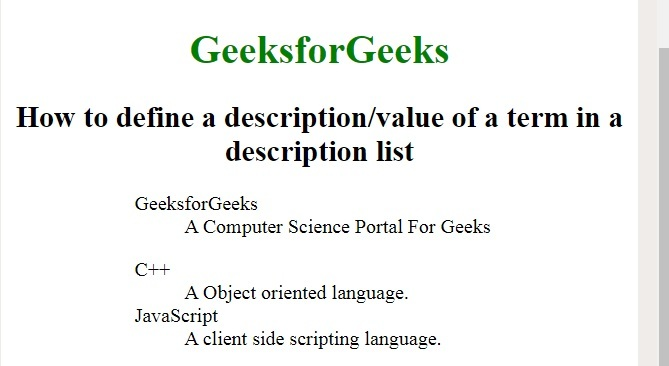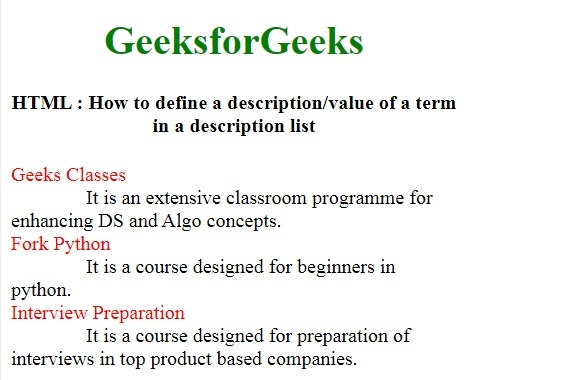# How to add description list of an element using HTML ?

To define the description of an element, the <dl> tag is used. This tag is used with <dt> and <dd> tag. In HTML4.1, it defines the definition list and in HTML5, it defines the description list.

Syntax:

`<dl> Contents... </dl>`

Example 1:

 ` ` `<``html``> ` ` `  `<``head``> ` `    ``<``title``> ` `        ``How to add description list ` `        ``of an element using HTML? ` `    `` ` ` `  `    ``<``style``> ` `        ``h1, ` `        ``h2 { ` `            ``text-align: center; ` `        ``} ` ` `  `        ``h1 { ` `            ``color: green; ` `        ``} ` ` `  `        ``dl { ` `            ``margin-left: 20%; ` `        ``} ` `    `` ` ` ` ` `  `<``body``> ` `    ``<``h1``>GeeksforGeeks ` ` `  `    ``<``h2``> ` `        ``How to define a description/value ` `        ``of a term in a description list ` `    `` ` ` `  `    ``<``dl``> ` `        ``<``dt``>GeeksforGeeks ` `        ``<``dd``>A Computer Science Portal For Geeks ` `    `` ` `     `  `    ``<``dl``> ` `        ``<``dt``>C++ ` `        ``<``dd``>A Object oriented language. ` `        ``<``dt``>JavaScript ` `        ``<``dd``>A client side scripting language. ` `    `` ` ` ` ` `  ` `

Output:Example 2:

 ` ` `<``html``> ` ` `  `<``head``> ` `    ``<``title``> ` `        ``How to add description list ` `        ``of an element using HTML? ` `    `` ` ` `  `    ``<``style``> ` `        ``h1 { ` `            ``color: green; ` `        ``} ` ` `  `        ``h1, ` `        ``h2 { ` `            ``text-align: center; ` `        ``} ` ` `  `        ``body { ` `            ``width: 70%; ` `        ``} ` ` `  `        ``dt { ` `            ``color: red; ` `        ``} ` ` `  `        ``dd { ` `            ``display: inline; ` `            ``margin-left: 60px; ` `        ``} ` `    `` ` ` ` ` `  `<``body``> ` `    ``<``h1``>GeeksforGeeks ` `    ``<``center``> ` `        ``<``h4``> ` `            ``How to define a description/value ` `            ``of a term in a description list ` `        `` ` `    `` ` ` `  `    ``<``dl``> ` `        ``<``dt``>Geeks Classes ` `        ``<``dd``> ` `            ``It is an extensive classroom programme ` `            ``for enhancing DS and Algo concepts. ` `        `` ` `        ``<``br` `/> ` ` `  `        ``<``dt``>Fork Python ` `        ``<``dd``> ` `            ``It is a course designed for  ` `            ``beginners in python. ` `        `` ` ` `  `        ``<``br` `/> ` `        ``<``dt``>Interview Preparation ` `        ``<``dd``> ` `            ``It is a course designed for  ` `            ``preparation of interviews in  ` `            ``top product based companies. ` `        `` ` `    `` ` ` ` ` `  ` `

Output:Supported Browsers: